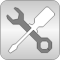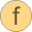# Utilities

Utility models to construct fluid components (should not be used directly)# Package ContentscheckBoundary Check whether boundary definition is correctregRoot Anti-symmetric square root approximation with finite derivative in the originregRoot_der Derivative of regRootregSquare Anti-symmetric square approximation with non-zero derivative in the originregPow Anti-symmetric power approximation with non-zero derivative in the originregRoot2 Anti-symmetric approximation of square root with discontinuous factor so that the first derivative is finite and continuousregSquare2 Anti-symmetric approximation of square with discontinuous factor so that the first derivative is non-zero and is continuousregStep Approximation of a general step, such that the characteristic is continuous and differentiableevaluatePoly3_derivativeAtZero Evaluate polynomial of order 3 that passes the origin with a predefined derivativeregFun3 Co-monotonic and C1 smooth regularization functioncubicHermite Evaluate a cubic Hermite splinecubicHermite_withDerivative Evaluate a cubic Hermite spline, return value and derivative